Courses

# Past Year Questions: Stress And Strain

## 23 Questions MCQ Test Engineering Mechanics | Past Year Questions: Stress And Strain

Description
This mock test of Past Year Questions: Stress And Strain for Mechanical Engineering helps you for every Mechanical Engineering entrance exam. This contains 23 Multiple Choice Questions for Mechanical Engineering Past Year Questions: Stress And Strain (mcq) to study with solutions a complete question bank. The solved questions answers in this Past Year Questions: Stress And Strain quiz give you a good mix of easy questions and tough questions. Mechanical Engineering students definitely take this Past Year Questions: Stress And Strain exercise for a better result in the exam. You can find other Past Year Questions: Stress And Strain extra questions, long questions & short questions for Mechanical Engineering on EduRev as well by searching above.
QUESTION: 1

### Two identical circular rods of same diameter and same length are subjected to same magnitude of axial tensile force. One of the rods is made out of mild steel having the modulus of elasticity of 206 GPa. The other rod is made out of east iron having the modulus of elasticity of 100 GPa. Assume both the materials to be homogeneous and isotropic and the axial force causes the same amount of uniform stress in both the rods. The stresses developed are within the proportional limit of the respective materials. Which of the following observations is correct? 

Solution:

Emild steel = 206 GPa
Ecast iron = 100 GPa
Now, Elongation in cast iron,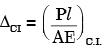Elongation in mild steel,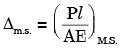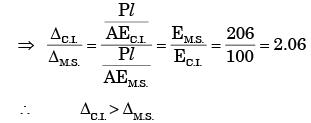QUESTION: 2

### A rod of length L having uniform cross-section area A is subjected to a tensile force P as shown in the figure below. If the Young's modulus of the material varies linearly from E1 to E2 along the length of the rod, the normal stress developed at the section-SS at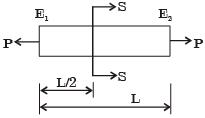Solution:

At section 55 : -
The left side of rod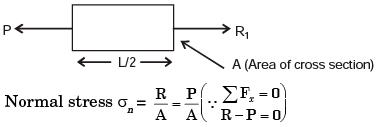The right side of rod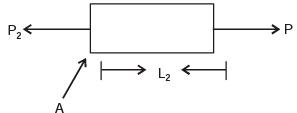∑ fx = 0
⇒ R2 – P = 0
⇒ R2 = P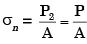∴ Hence normal = P/A

QUESTION: 3

### A thin plate of uniform thickness is subject to pressure as shown in the figure below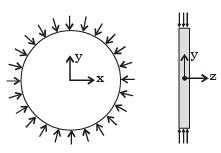Under the assumption of plane stress, which one of the following is correct? 

Solution:

For a plane stress criteria.
Normal stress in Z direction = 0.

QUESTION: 4

The stress-strain curve for mild steel is shown in figure given below. Choose the correct option referring to both figure and table.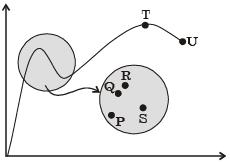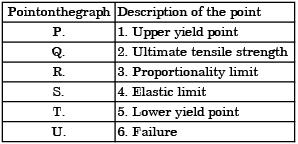Solution:
QUESTION: 5

Which one of the following types of stress-strain relationship best describes the behavior of brittle materials, such as ceramics and thermosetting plastics, (σ = stress and ε - strain)?



Solution:

Brittle material breaks without plastic deformation.

QUESTION: 6

A rod of length 20 mm is stretched to make a rod of length 40 mm. Subsequently, it is compressed to make a rod of final length 10 mm. Consider the longitudinal tensile strain as positive and compressive strain as negative.The total true longitudinal strain in the rod is



Solution:

Volume remain same
L1 = 20 mm, L2 = 40 mm, L3 = 10mm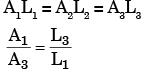True strain =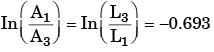QUESTION: 7

In the engineering stress-strain curve for mild steel, the Ultimate Tensile Strength (UTS) refers to



Solution:
QUESTION: 8

The Poisson's ratio for a perfectly incompressible linear elastic material is



Solution:
QUESTION: 9

Below figure shows a rigid bar hinged at A and supported in a horizontal position by two vertical identical steel wires. Neglect the weight of the beam. The tension T1 and T2 induced in these wires by a vertical load P applied as shown are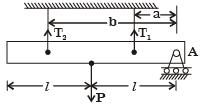Solution: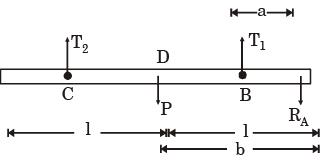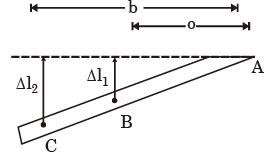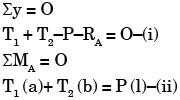Using similar Δ,s in fig (2)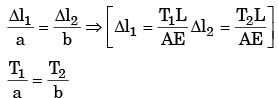Putting above eqn in eqn (ii)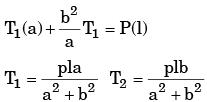QUESTION: 10

In terms of Poisson's ratio (μ) the ratio of Young's modulus (E) to Shear modulus (G) of elastic materials is



Solution:

We know
E = 2G[1 + v]
E/G = 2[1 + v].

QUESTION: 11

A 200 × 100 × 50 mm steel block is subjected to a hydrostatic pressure of 15 MPa. The Young's modulus and Poisson's ratio of the material are 200 GPa and 0.3 respectively. The change in the volume of the block in mm3 is



Solution:

Here, Px = Py = Pz = – 15 × 106 Pa (compression)
Now,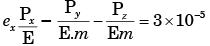where, m = Poisson’s ratio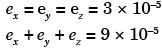∴ Change in volume = Total strain × Original = 90 m3

QUESTION: 12

A rod of length L and diameter D is subjected to a tensile load P. Which of the following is sufficient to calculate the resulting change in diameter?



Solution:
QUESTION: 13

A cylindrical container of radius R = 1 m, wall thickness 1 mm is filled with water up to a depth of 2 m and suspended along its upper rim. The density of water is 1000 kg/m3 and acceleration due to gravity is 10 m/s2. The self-weight of the cylinder is negligible. The formula for hoop stress in a thin-walled cylinder can be used at all points along the height of the cylindrical container.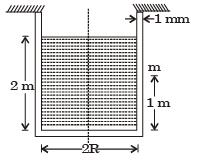The axial and circumferential stress (σa, σc) experienced by the cylinder wall at mid-depth (m as shown) are



Solution:

Pressure at mid – depth = ρgh
= 103 × 10 × 1 = 104 N /m2
∴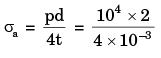= 5 × 106 N/mm2 = 5 MPa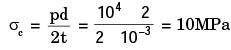QUESTION: 14

A cylindrical container of radius R = 1 m, wall thickness 1 mm is filled with water up to a depth of 2 m and suspended along its upper rim. The density of water is 1000 kg/m3 and acceleration due to gravity is 10 m/s2. The self-weight of the cylinder is negligible. The formula for hoop stress in a thin-walled cylinder can be used at all points along the height of the cylindrical container.If the Young's modulus and Poisson's ratio of the container material' are 100 GPa and 0.3, respectively, the axial strain in the cylinder wall at mid-depth is



Solution:

Axial strain in the cylinder would be produced due to the axial (longitudinal) stress and due to the lateral stress i .e. hoop stress, e =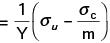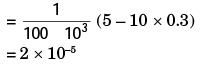QUESTION: 15

The figure below shows a steel rod of 25 mm2 cross sectional area. It is loaded at four points. K, L, M and N. Assume Esteel = 200 GPa. The total change in length of the rod due to loading is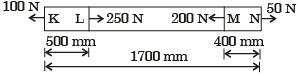Solution: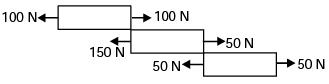Total change in length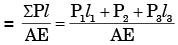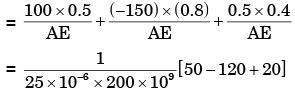=  –1 × 10–5 m = –10μm

QUESTION: 16

A bar having a cross-sectional area of 700 mm2 is subjected to axial loads at the positions indicated. The value of stress in the segment QR is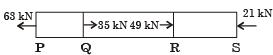Solution: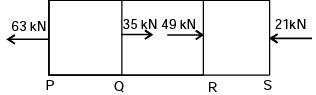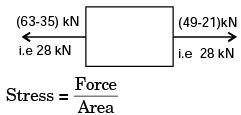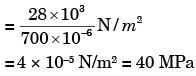QUESTION: 17

A bimetallic cylindrical bar of cross sectional area 1 m2 is made by bonding Steel (Young's modulus = 210 GPa) and Aluminium (Young's modulus = 70 GPa) as shown in the figure. To maintain tensile axial strain of magnitude 10–6 in steel bar and compressive axial strain of magnitude 10–6 in Aluminum bar, the magnitude of the required force P(in kN) along the indicated direction is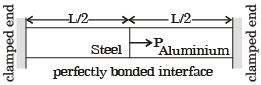Solution: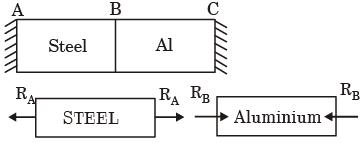Let RA & RB be the reation at the supports A & B. For the equilibrium of the bar these reaction must act towards left. so that;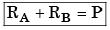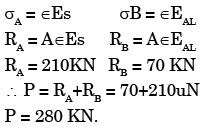QUESTION: 18

A free bar of length / is uniformly heated from 0°C to a temperature t°C, α is the coefficient of linear expansion and E the modulus of elasticity. The stress in the bar is



Solution:

For thermal stress to be devel oped there must be constraint in the system to oppose. So strain develops but there is no thermal stress.

QUESTION: 19

A uniform, slender cylindrical rod is made of a homogeneous and isotropic material. The rod rests on a frictionless surface. The rod is heated uniformly. If the radial and longitudinal thermal stresses are represented by σr and σz respectively, then



Solution:

Rod is not restrained but completely free

QUESTION: 20

A steel rod of length L and diameter D, fixed at both ends, is uniformly heated to a temperature rise of ΔT. The Young's modulus is E and the coefficient of linear expansion is a. The thermal stress in the rod is



Solution:

Since rod is free to expand, therefore
ΔL = elongation = LαΔT
∴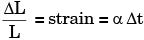Thermal stress = E α Δt
Since rod is fixed at both ends, so thermal strain will be zero but there will be thermal stresses.

QUESTION: 21

A solid steel cube constrained on all six faces is heated so that the temperature rises uniformly by ΔT. If the thermal coefficient of the material is α, Young's modulus is E and the Poisson's ratio is v , the thermal stress developed in the cube due to heating is



Solution:

Volumetric strain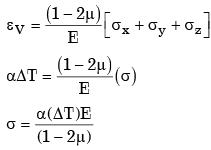QUESTION: 22

A circular rod of length L and area of crosssection A has a modulus of elasticity E and coefficient of thermal expansion α. One end of the rod is fixed and other end is free. If the temperature of the rod is increased by ΔT, then



Solution:

Since one end of the rod is fixed and other is free to expand. Hence the temperature stresses is zero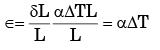QUESTION: 23

A steel cube, with all faces free to deform, has Young's modulus, E, Poisson's ratio, v, and coefficient of thermal expansion, a. The pressure (hydrostatic stress) developed within the cube, when it is subjected to a uniform increase in temperature, ΔT, is given by



Solution:

Since all the faces are free to expand the stresses due to temperature rise is equal to 0.